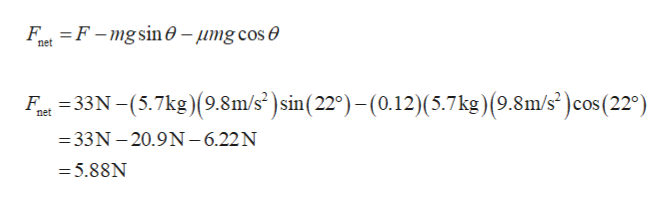# m

Question
7 views

A block of mass m = 5.7 kg is pulled up a θ = 22° incline as in the figure below with a force of magnitude F = 33 N.

Find the acceleration of the block if the incline is frictionless. (Give the magnitude of the acceleration.)

Find the acceleration of the block if the coefficient of kinetic friction between the block and incline is 0.12. (Give the magnitude of the acceleration.)

check_circle

Step 1

(a)

Consider the surface is frictionless, and then the net force acting along the inclined plane is.

Substitute the values,

Step 2

According to the Newton’s second law, find the acceleration of the block.

Substitute the values,

Step 3

(b)

Consider the surface is acting now the friction force, then the ne...help_outlineImage TranscriptioncloseFt F-mgsin 0-umg cos e net at335.7kg)(9.8m/s)sin(220)-(0.12)(5.7 kg) (9.8m/s) cos (220) net =33N -20.9N-6.22N = 5.88N fullscreen

### Want to see the full answer?

See Solution

#### Want to see this answer and more?

Solutions are written by subject experts who are available 24/7. Questions are typically answered within 1 hour.*

See Solution
*Response times may vary by subject and question.
Tagged in

### Newtons Laws of Motion